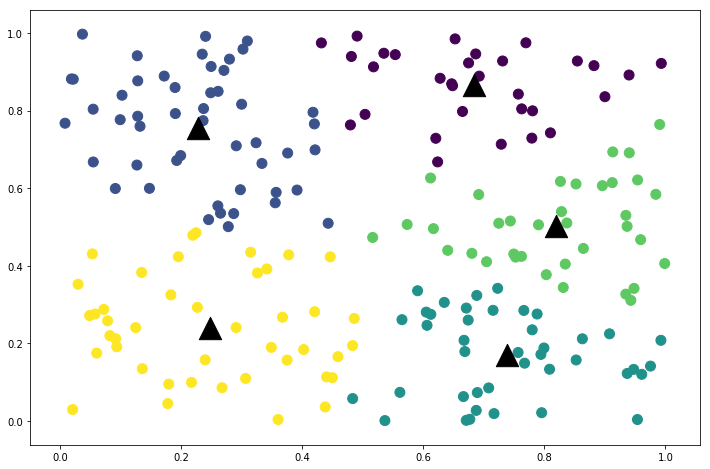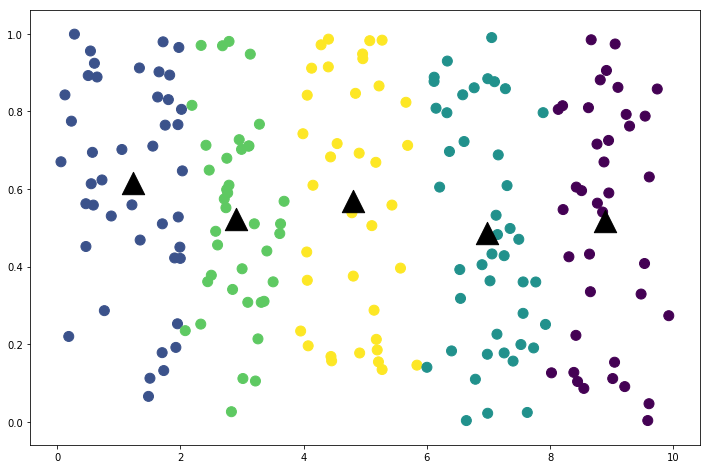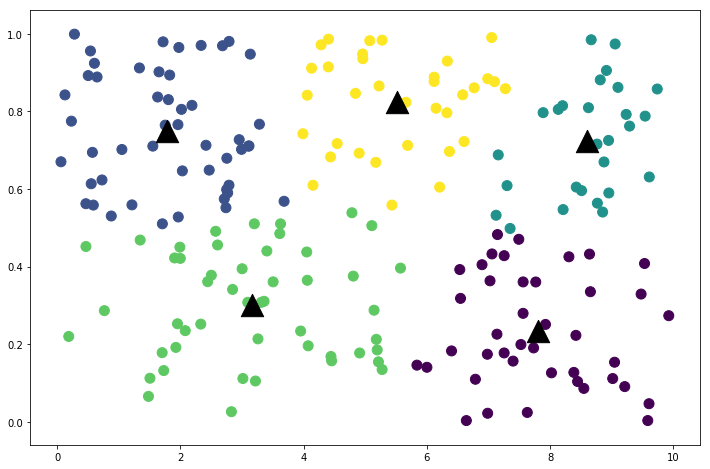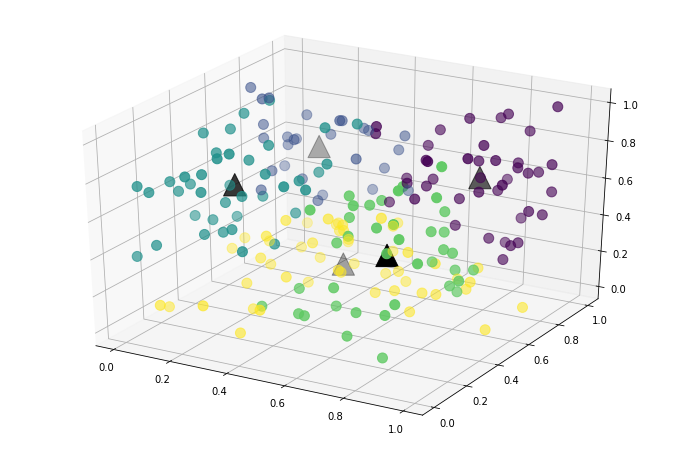## 使用numpy高效实现 k-means聚类

2020年12月07日

K-Means原理很简单。按照西瓜书的定义，就是在给定样本集 $D={x_{1},x_{2},…,x_{m}}$，找出簇中心$C={C_{1}, C_{2}…C{k}}$来最小化平方误差：

$E=\sum_{i=1}^{k}\sum_{x\in{C_{i}}}\left|\right|\vec{x}-\vec{u_{i}}\left|\right|^2$

def kmeans(data, k=3, normalize=False, limit=500):
# normalize 数据
if normalize:
stats = (data.mean(axis=0), data.std(axis=0))
data = (data - stats) / stats

# 直接将前K个数据当成簇中心
centers = data[:k]

for i in range(limit):
# 首先利用广播机制计算每个样本到簇中心的距离，之后根据最小距离重新归类
classifications = np.argmin(((data[:, :, None] - centers.T[None, :, :])**2).sum(axis=1), axis=1)
# 对每个新的簇计算簇中心
new_centers = np.array([data[classifications == j, :].mean(axis=0) for j in range(k)])

# 簇中心不再移动的话，结束循环
if (new_centers == centers).all():
break
else:
centers = new_centers
else:
# 如果在for循环里正常结束，下面不会执行
raise RuntimeError(f"Clustering algorithm did not complete within {limit} iterations")

# 如果normalize了数据，簇中心会反向 scaled 到原来大小
if normalize:
centers = centers * stats + stats

return classifications, centers


data = np.random.rand(200, 2)
classifications, centers = kmeans(data, k=5)


from mpl_toolkits.mplot3d import Axes3D
import matplotlib.pyplot as plt

%matplotlib inline

plt.figure(figsize=(12, 8))
plt.scatter(x=data[:, 0], y=data[:, 1], s=100, c=classifications)
plt.scatter(x=centers[:, 0], y=centers[:, 1], s=500, c='k', marker='^');
# 16轮迭代结束k-means算法对每个数据维数的相对尺度很敏感。如果某个维度是更大，距离函数会在该维度的计算权重会更大一些。直观一些，当我们将数据第一维扩大10倍后，观察一下：

data = np.random.rand(200, 2)
data[:, 0] *= 10  # 第一维扩大10倍

classifications, centers = kmeans(data, k=5)

plt.figure(figsize=(12, 8))
plt.scatter(x=data[:, 0], y=data[:, 1], s=100, c=classifications)
plt.scatter(x=centers[:, 0], y=centers[:, 1], s=500, c='k', marker='^');
# 13轮迭代结束classifications, centers = kmeans(data, normalize=True, k=5)

plt.figure(figsize=(12, 8))
plt.scatter(x=data[:, 0], y=data[:, 1], s=100, c=classifications)
plt.scatter(x=centers[:, 0], y=centers[:, 1], s=500, c='k', marker='^');
# 9轮迭代结束data = np.random.rand(200, 3)

classifications, centers = kmeans(data, normalize=True, k=5)

fig = plt.figure(figsize=(12, 8))